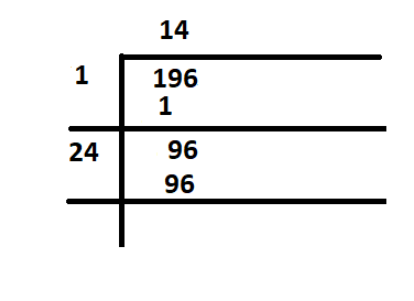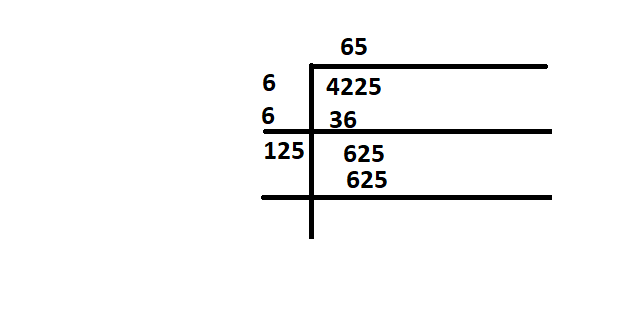Open in App
Not now

# How to find the square roots of 196?

• Last Updated : 03 Sep, 2021

The arithmetic value which is used for representing the quantity and used in making calculations are defined as Numbers. A symbol like “4, 5, 6” which represents a number is known as numerals. Without numbers, counting things is not possible, date, time, money, etc. these numbers are also used for measurement and used for labeling. The properties of numbers make them helpful in performing arithmetic operations on them. These numbers can be written in numeric forms and also in words.

For example, 3 is written as three in words, 35 is written as thirty-five in words, etc. Students can write the numbers from 1 to 100 in words to learn more. There are different types of numbers, which we can learn. They are whole and natural numbers, odd and even numbers, rational and irrational numbers, etc

### Number System

A Number System is a method of showing numbers by writing, which is a mathematical way of representing the numbers of a given set, by using the numbers or symbols in a mathematical manner. The writing system for denoting numbers using digits or symbols in a logical manner is defined as Number System. The digits from 0 to 9 form all the numbers. With these digits, anyone can create infinite numbers. For example, 156,3907, 3456, 1298, 784859, etc

### Square root

The value of a number of square roots, which on multiplication by itself gives the original number. Suppose, a is the square root of b, then it is represented as a = √b or we can express the same equation as a2 = b. Here,’√’ this symbol we used to represent the root of numbers is termed as radical. The positive number when it is to be multiplied by itself represents the square of the number. The square root of the square of any positive number gives the original number.

For example, the square of 4 is 16, 42 = 16, and the square root of 16, √16 = 4. Since 4 is a perfect square and we have both the negative and positive square root value of 16 is ±4,means 16 has two square roots one is 4 and second is -4, because 4 x 4 = -4 x -4 = 16. Hence it is easy to find the square root of such numbers, but for an imperfect square, it’s really tricky.

Square Root is represented as ‘√’. It is called a radical symbol. To represent a number ‘a’ as a square root using this symbol can be written as: ‘√a‘, where a is the number. The number here under the radical symbol is called the radicand. For example, the square root of 4 is also represented as a radical of 4. Both represent the same value, and the formula to find the square root is: b = √a

Properties of Square Roots

It is defined as a one-to-one function that takes a positive number as an input and returns the square root of the given input number. For example, here if x = 9, then the function returns the output value as 3.

f(x) = √x

• If a number is a perfect square number, then there definitely exists a perfect square root.
• If a number ends with an even number of zeros (0’s), then we can have a square root.
• The two square root values can be multiplied. For example, √3 can be multiplied by √2, then the result will be √6.
• When two same square roots are multiplied, then the result must be a radical number. It shows that the result is a non-square root number. For example, when √7 is multiplied by √7, the result obtained is 7.
• The square root of negative numbers is undefined. hence the perfect square cannot be negative.
• Some of the numbers end with 2, 3, 7, or 8 (in the unit digit), then the perfect square root does not exist.
• Some of the numbers end with 1, 4, 5, 6, or 9 in the unit digit, then the number will have a square root.

It is easy to find the square root of a number that is a perfect square.

Perfect squares

Perfect squares are those positive numbers or negative numbers that can be written as the multiplication of a number by itself, or you can say that a perfect square is a number which is the value of power 2 of any integer. The number can be expressed as the product of two equal integers. For example, 16 is a perfect square because it is the product of two equal integers, 4 × 4 = 16. or -4 × -4 = 16, However, 24 is not a perfect square because it cannot be expressed as the product of two equal integers. (8 × 3 = 24).

The number which is obtained by squaring a whole number is termed as a Perfect square.

Assume N is a perfect square of a whole number y, this can be written as N = the product of y and y = y2. So, the perfect square formula can be expressed as,

N = Y2

Let’s Use the formula with values.

If y = 5, and N = y2

This means, N = 52 = 25 or (- 5)2= 25

Here, 5 is the positive and negative square root of 25 i.e ±5

therefore there are two square roots of 25: 5 or -5

### How to find the square roots of 196?

With the help of square roots, It is easy to identify whether a number is a perfect square or not. If the square root is a whole number then the given number will be a perfect square, and if the square root value is not a whole number, then the given number is not a perfect square.

Solution:Here square root of 196 is 14, which can be written as ±14,

So, 14 and -14 are the two square roots of 19, n its a perfect square of 196.

And √196 = 14 or 142 = 14 × 14 = 196

Same √196 = 14 or (-14)2= -14 × -14 = 196.

### Similar Problems

Question 1: What are the two square roots of 4225?

Solution:square root of 4225

Here 65 is the square root of 4225

±65 are the square root of 4225

So 65 and – 65 are the two square root of 4225.

Question 2: what are the two square roots of 900?

Solution:

Here 900 is the perfect square of 30

302 = 30 × 30 = 900

Or  we can write as (-30)2 = (-30) × (-30) = 900

So 30 and -30 are the two square roots of 900.

i.e √900 = ±30

My Personal Notes arrow_drop_up